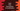# Java program to find Permutation and Combination ( nPr and nCr )### Java program to find Permutation and Combination ( nPr and nCr ) of two numbers :

In this example, we will learn how to find permutation and combination of two numbers. Permutation is denoted as nPr and combination is denoted as nCr. nPr means permutation of ‘n’ and ‘r’. nCr means combination of ‘n’ and ‘r’.

``````nPr = factorial(n) / factorial(n-r)
nCr = factorial(n)/(factorial(n-r) * factorial(r))
``````

So, we need only one factorial utility function to calculate values of nPr and nCr. We will get the value of ‘n’ and ‘r’ from the user and calculate the values. Then we will print out both valus.

### Example Program :

``````import java.util.Scanner;

public class Main {

/**
* Utility functions for System.out.println() and System.out.print()
*/
private static void print(String str) {
System.out.print(str);
}

private static void println(String str) {
System.out.println(str);
}

/**
* Find the factorial of a number
*
* @param n : number to find the factorial
* @return : factorial value of 'n'
*/
public static int factorial(int n) {
//1
int fact = 1;
//2
for (int i = 1; i <= n; i++) {
//3
fact = fact * i;
}
//4
return fact;
}

public static void main(String args[]) {
int n, r;
Scanner scan = new Scanner(System.in);

println("To calculate the nCr and nPr values, we need 'n' and 'r' value .");

print("Enter the value of n : ");
n = scan.nextInt();

print("Enter the value of r : ");
r = scan.nextInt();

int ncr = (factorial(n) / (factorial(n - r) * factorial(r)));

int npr = (factorial(n) / (factorial(n - r)));

println("nPr is : " + npr);
println("nCr is : " + ncr);
}

}
``````

### Sample Output :

``````To calculate the nCr and nPr values, we need 'n' and 'r' value .
Enter the value of n : 11
Enter the value of r : 10
nPr is : 39916800
nCr is : 11

To calculate the nCr and nPr values, we need 'n' and 'r' value .
Enter the value of n : 12
Enter the value of r : 12
nPr is : 479001600
nCr is : 1
``````

### How the factorial() function works :

The commented numbers in the above program denote the steps number below :

1. public static int factorial(int n) method takes one integer ‘n’ as input and returns one integer (factorial value of n) after the completion. Inside the function, we have first initialized one integer variable ’fact’ to store the final factorial value. The value of ‘fact’ is ‘1’ at start.

2. Now, start one ’for loop’. This loop will run from_ ‘i = 1’ to ‘i = n’_ . So, if we are calculating the factorial value of ’10’, it will run for _‘i=1’, ‘i=2’…‘i=10’. _

3. Inside the loop, multiply the number with ‘fact’. For example, for value ‘10’, :

``````1. _ For 'n=1', fact = fact * n = 1 * 1 = 1_

2. _ For 'n=2', fact = fact * n = 1 * 2 = 2_

3. _ For 'n=3', fact = fact * n = 2 * 3 = 6_

4. _ For 'n=4', fact = fact * n = 6 * 4 = 24_ and so on.``````
1. So , after the ’for loop’ is completed, ’fact’ will hold the value of ’1 * 2 * 3 * 4 * 5 * 6 * 7 * 8 * 9 * 10’ i.e. factorial 10. Now return this value to the main function.

In this way, we can calculate the factorial of ‘n’, ‘n-r’ and ‘r’ to find the value of ’nPr’ and ’nCr‘.